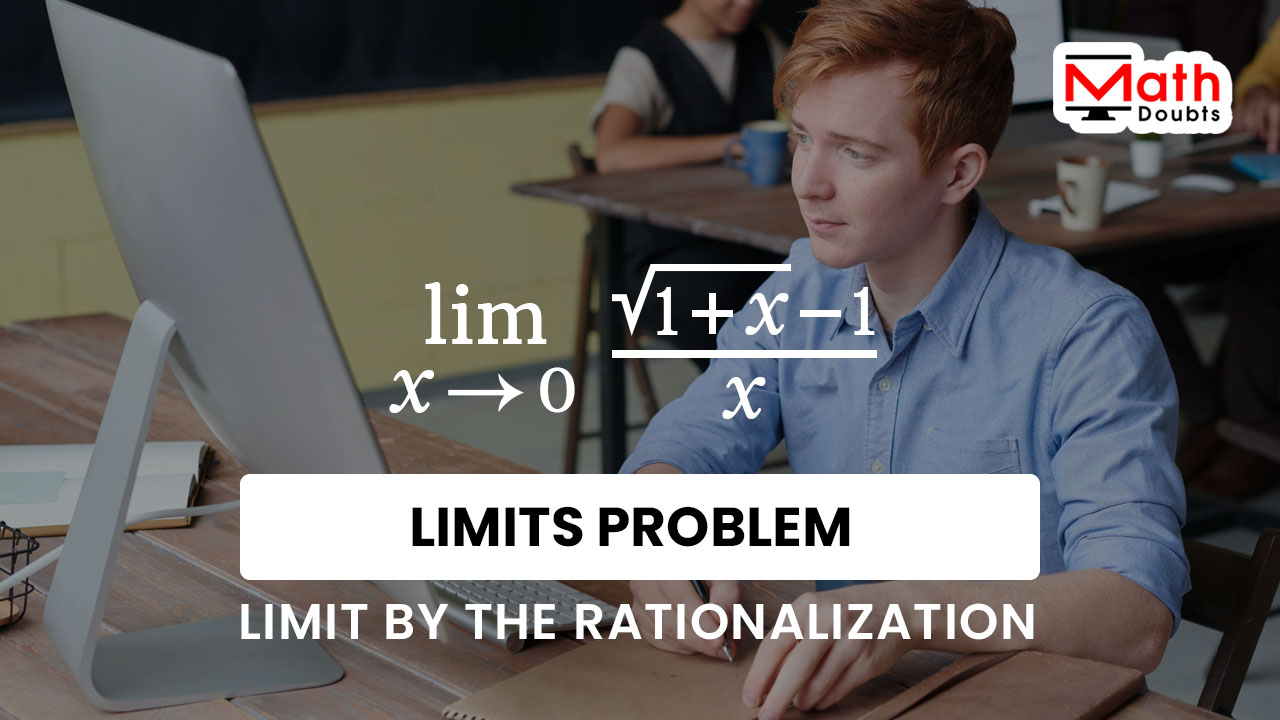# Evaluate $\displaystyle \large \lim_{x\,\to\,0}{\normalsize \dfrac{\sqrt{1+x}-1}{x}}$ by Rationalization

The limit of square root of one plus $x$ minus one divided by $x$ is indeterminate as the value of $x$ approaches zero as per the direct substitution method.

$\displaystyle \large \lim_{x\,\to\,0}{\normalsize \dfrac{\sqrt{1+x}-1}{x}}$ $\,=\,$ $\dfrac{0}{0}$It is given in this limit question that the limit of square root of $1$ plus $x$ minus $1$ divided by $x$ should be evaluated by rationalization as the value of $x$ tends to $0$.

### Remove the indeterminate form by Rationalisation

An expression in radical form the square root of $1$ plus $x$ is involved in forming the function in the numerator. So, let us try to remove the irrational form of the expression in the numerator by rationalizing it with its conjugate function.

$=\,\,$ $\displaystyle \large \lim_{x\,\to\,0}{\normalsize \bigg(\dfrac{\sqrt{1+x}-1}{x}}$ $\times$ $1\bigg)$

$=\,\,$ $\displaystyle \large \lim_{x\,\to\,0}{\normalsize \bigg(\dfrac{\sqrt{1+x}-1}{x}}$ $\times$ $\dfrac{\sqrt{1+x}+1}{\sqrt{1+x}+1}\bigg)$

### Find the Product by simplifying Rational function

Now, it is time to multiply the two rational expressions consisting irrational form expressions by the multiplication rule of fractions.

$=\,\,$ $\displaystyle \large \lim_{x\,\to\,0}{\normalsize \dfrac{\big(\sqrt{1+x}-1\big) \times \big(\sqrt{1+x}+1\big)}{x \times \big(\sqrt{1+x}+1\big)}}$

The product of the functions in the numerator can be multiplied by the difference of squares formula.

$=\,\,$ $\displaystyle \large \lim_{x\,\to\,0}{\normalsize \dfrac{\big(\sqrt{1+x}\big)^2-1^2}{x\big(\sqrt{1+x}+1\big)}}$

Now, let us focus on simplifying the expressions in both numerator and denominator of the rational function.

$=\,\,$ $\displaystyle \large \lim_{x\,\to\,0}{\normalsize \dfrac{1+x-1}{x\big(\sqrt{1+x}+1\big)}}$

$=\,\,$ $\displaystyle \large \lim_{x\,\to\,0}{\normalsize \dfrac{\cancel{1}+x-\cancel{1}}{x\big(\sqrt{1+x}+1\big)}}$

$=\,\,$ $\displaystyle \large \lim_{x\,\to\,0}{\normalsize \dfrac{x}{x\big(\sqrt{1+x}+1\big)}}$

$=\,\,$ $\displaystyle \large \lim_{x\,\to\,0}{\normalsize \dfrac{\cancel{x}}{\cancel{x}\big(\sqrt{1+x}+1\big)}}$

$=\,\,$ $\displaystyle \large \lim_{x\,\to\,0}{\normalsize \dfrac{1}{\sqrt{1+x}+1}}$

### Evaluate the Limit by Direct substitution

Now, let us find the limit of the reciprocal of square root of one plus $x$ plus one by substituting $x$ is equal to zero directly.

$=\,\,$ $\dfrac{1}{\sqrt{1+0}+1}$

$=\,\,$ $\dfrac{1}{\sqrt{1}+1}$

$=\,\,$ $\dfrac{1}{1+1}$

$=\,\,$ $\dfrac{1}{2}$

Latest Math Topics
Jun 26, 2023
Jun 23, 2023

Latest Math Problems
Jul 01, 2023
Jun 25, 2023
###### Math Questions

The math problems with solutions to learn how to solve a problem.

Learn solutions

Practice now

###### Math Videos

The math videos tutorials with visual graphics to learn every concept.

Watch now

###### Subscribe us

Get the latest math updates from the Math Doubts by subscribing us.